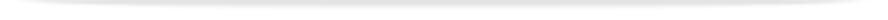units

MTH2015

Faculty of Science

This unit entry is for students who completed this unit in 2014 only. For students planning to study the unit, please refer to the unit indexes in the the current edition of the Handbook. If you have any queries contact the managing faculty for your course or area of study.print version

## 6 points, SCA Band 2, 0.125 EFTSL

Refer to the specific census and withdrawal dates for the semester(s) in which this unit is offered, or view unit timetables.

 Level Undergraduate Faculty Faculty of Science Organisational Unit School of Mathematical Sciences Monash Passport category Advanced Studies (Enhance Program) Offered Clayton Second semester 2014 (Day) Coordinator(s) Mr John McCloughan

### Synopsis

This unit is an alternative to MTH2010 for students with a strong mathematical foundation.
Functions of several variables, partial derivatives, extreme values, Lagrange multipliers. Multiple integrals, line integrals, surface integrals. Vector differential calculus; grad, div and curl. Integral theorems of Gauss and Stokes. Use of a computer algebra package.

### Outcomes

On completion of this unit students will be able to:

1. Understand and apply multivariable calculus to problems in the mathematical and physical sciences;

1. Find and classify the extrema of functions of several variables;

1. Compute Taylor series for functions of several variables;

1. Compute line, surface and volume integrals in Cartesian, cylindrical and polar coordinates;

1. Apply the integral theorems of Green, Gauss and Stokes;

1. Use computer algebra packages to solve mathematical problems;

1. Present a mathematical argument in written form;

1. Understand and apply the formal definition of a limit to functions of several variables;

1. Prove various identities between grad, div and curl;

1. Develop and present rigorous mathematical proofs.

### Assessment

Continuous assessments: 40%
Final Examination: 60%

### Chief examiner(s)